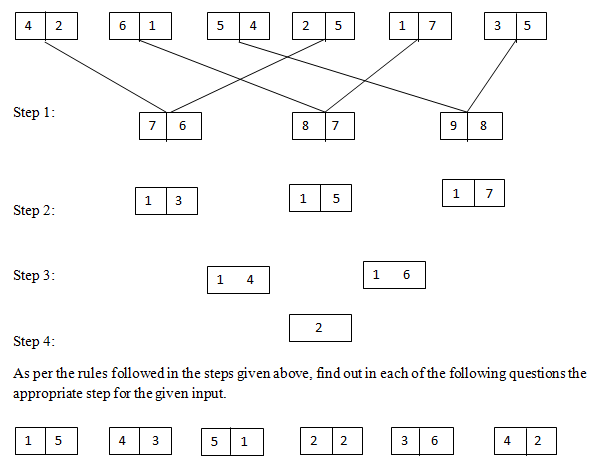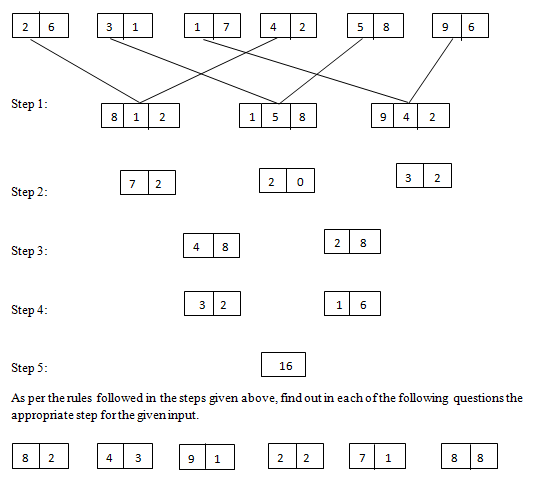# Reasoning – New Pattern Input Output Questions Day-3

Dear Readers, We all knew that IBPS has introduced some new pattern Reasoning Questions in the Examinations. Many of our followers were requesting us to provide Practice Questions based on the new pattern, so for that we have started providing input and output reasoning questions. Kindly follow us regularly and make use of it, if you have any suggestion kindly use the comment section below.

[WpProQuiz 745]

### Click “Start Quiz” to attend these Questions and view Solutions

Directions (1-5): Study the given information carefully and answer the given questions.

An input-output is given in different steps. Some mathematical operations are done in each step. No mathematical operation is repeated in next step.1) Which of the following is the sum of 1st digit of the 3rd block and 2nd digit of the second block in step 2?

a)7

b)9

c)10

d)4

e)None of these

2) What is the 50% value of the first block in step 3?

a)26

b)19

c)24

d)18

e) None of these

3) If the second block of step 2 is divided by the final output, then what will be the resultant value?

a)1

b)2

c)3

d)1.5

e) None of these

4) If all the digits in step 2 is added, then multiplied with final step, what will be the answer?

a)225

b)289

c)256

d)196

e) None of these

5) If the 3rd block of 1st step is divided by 3 and multiplied with 7, then what will be the answer?

a)91

b)84

c)98

d)86

e) None of these

Directions (6-10): Study the given information carefully and answer the given questions.

An input-output is given in different steps. Some mathematical operations are done in each step. No mathematical operation is repeated in next step.6) If the sum of 1st digit of 3rd block and 3rd digit of 1st block in step1 is multiplied with 2, then what will be the answer?

a)16

b)24

c)22

d)18

e)None of these

7) If the value of 2nd block in step 3 is divided by the 1st block in step 2, then what will be the resultant value?

a)6

b)7

c)8

d)9

e)5

8) which of the following represents the sum of 1st digit in 1st block and 2nd digit in 2nd block in step 3?

a)13

b)17

c)11

d)18

e)16

9)If ‘4’ is added with each digits in step 4, then what will be the product of values in 1st block?

a)77

b)44

c)42

d)63

e)66

10) What will be the difference between 1st block in step 3 and final step?

a)18

b)20

c)24

d)23

e)None of these

• #### Reasoning Input Output New Pattern Questions – Day-2